>  Chemistry Test - 6

# Chemistry Test - 6

Test Description

## 30 Questions MCQ Test | Chemistry Test - 6

 1 Crore+ students have signed up on EduRev. Have you?
Chemistry Test - 6 - Question 1

### Of the following acids I. Hypophosphorous acid II. Orthophosphorous acid III. Caro’s acid IV. Glycine

Chemistry Test - 6 - Question 2

### Cationic addition polymerisation reaction initiated by an acid is maximum in

Chemistry Test - 6 - Question 3

### Magnetic moments of Cr(Z = 24), Mn(Z = 25) and Fe(Z = 26) are x, y and z respectively. Hence

Chemistry Test - 6 - Question 4

Silica is added to roasted copper ore during smelting in order to remove

Chemistry Test - 6 - Question 5

The reaction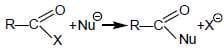is fastest when X is

Chemistry Test - 6 - Question 6

Which of the following pairs of structures is an example of diastereomers ?

Chemistry Test - 6 - Question 7

16 g of an ideal gas SOx occupies 5.6 L at STP. The value of x for this gas is

Chemistry Test - 6 - Question 8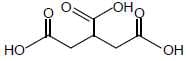. IUPAC name of the compound is

Chemistry Test - 6 - Question 9

When a certain conductivity cell was filled with 0.01 M solution of KCl, it had a resistance of 160 ohm at 250C and when filled with 0.005 M NaOH, it had a resistance of 190 ohm. If specific resistance of KCl solution is 700 ohm cm, specific conductance (ohm-1 cm-1) of NaOH solution is

Chemistry Test - 6 - Question 10

A buffer solution contains 100 mL of 0.01 M CH3COOH and 200 mL of 0.02 M CH2COONa. 700 mL of water is added to this solution . pH before and after dilution are (pKa = 4.74)

Chemistry Test - 6 - Question 11

Arrange the following compounds in decreasing order of basicity
I. ethylamine
II. 2-amino ethanol
III. 3-amino-1-propanol

Chemistry Test - 6 - Question 12

One gram of hydrogen and 112 g of nitrogen are enclosed in two separate containers each of volume 5 L at 270C. If the pressure of hydrogen is 1 atm, the pressure of nitrogen would be

Chemistry Test - 6 - Question 13

Ozonolysis products of an olefin are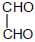and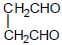Olefin is

Chemistry Test - 6 - Question 14

Following is the concentration cell in which electrode is reversible with respect to anion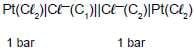. The cell reaction is spontaneous, if

Chemistry Test - 6 - Question 15

Consider the following reactions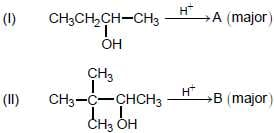A and B are respectively

Chemistry Test - 6 - Question 16

The correct name for the complex ion [Co(en)2Cl(ONO)]+ is

Chemistry Test - 6 - Question 17

A laboratory reagent imparts green colour to the flame. On heating with solid K2Cr2O7 and conc. H2SO4, it evolves an orange red gas. Identify the reagent.

Chemistry Test - 6 - Question 18

van’t Hoff factors are x, y and z in case of association, ionisation and no change, respectively. Their increasing order is

Chemistry Test - 6 - Question 19

Which of the following does not illustrate the anomalous properties of Li ?

Chemistry Test - 6 - Question 20

If for a reaction A → B, ΔH = -10 kJ mol-1 and Ea = 50 kJ mol-1, the energy of activation for reaction B → A will be

Chemistry Test - 6 - Question 21

NaAlH4 reduces an ester into HCHO and (CH3)2CHOH. Thus, ester is

Chemistry Test - 6 - Question 22

Reduction of a metal oxide by excess carbon at high temperature is a method for the commercial preparation of some metals. This method can be successfully applied in the case of

Chemistry Test - 6 - Question 23

What is the repeating unit in nucleic acids ?

Chemistry Test - 6 - Question 24

Elevation in boiling point of an aqueous urea solution is 0.520(kb = 0.520 mol-1 kg). Hence, mole fraction of urea in this solution is

Chemistry Test - 6 - Question 25

For the reaction, 2Fe3+ + Fe → 3Fe2+ , E0 = 1.21 V, hence

Chemistry Test - 6 - Question 26

A 100 mL sample is removed from water solution saturated with CaSO4 at 250C. The water is completely evaporated from the sample and a deposit of 0.24 g CaSO4 is obtained. The Ksp of CaSO4 at 250C is

Chemistry Test - 6 - Question 27

Match the following lists
List I                          List II
A. Benzene               1. Phosgene
B. Ethylene               2. Silver mirror
C. Acetaldehyde       3. Mustard gas
D. Chlororform          4. (4n + 2)π electrons
5. Carbylamine

Chemistry Test - 6 - Question 28

In a transition series, as the atomic number increases, paramagnetism

Chemistry Test - 6 - Question 29

When FeS2 is burnt in air, it converts to Fe2O3. The change in percentage by weight of iron in the process is (Fe = 56)

Chemistry Test - 6 - Question 30

Which of the following pairs of liquid form an emulsion ?

 Use Code STAYHOME200 and get INR 200 additional OFF Use Coupon Code